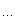# huaxiazhihuo

## 预处理的图灵完备之引言

#define PP_TEXT(str) _ZPP_TEXT(str)
#define _ZPP_TEXT(str) #str

#define PP_TEXT(str) #str

#define AAA aaa
PP_TEXT(AAA)，结果将是"AAA",而不是"aaa"。因为宏操作符直接是将入参变成字符串，没有让入参有一点点回旋的空间，所以只好引入间接层，让入参有机会宏展开。后面，很多宏函数都是这样实现，不得不间接调用，以便让宏全面展开。而msvc的宏展开机制更加奇葩，更加不人性化，其间接调用的形式也更丑陋。这都是没办法的事情。

#define PP_ASSERT() static_assert((__VA_ARGS__), PP_TEXT(__VA_ARGS__));
PP_ASSERT(...)里面的三个点，是不定参数的宏，而__VA_ARGS__就代表了...所匹配的所有参数，这条语法很重要，要熟练。这里，就不详细解释其用法了，后面会有大把大把的宏函数用到__VA_ARGS__。

PP_ASSERT(2+3==5)

PP_ASSERT(2+3==4)

#define PP_JOIN(_A, _B) _ZPP_JOIN_I(_A, _B)
#define _ZPP_JOIN_I(_A, _B) _ZPP_JOIN_II(~, _A##_B)
#define _ZPP_JOIN_II(p, res) res

PP_ASSERT(PP_JOIN(1+2== 3))
#define A 20
#define B 10
PP_ASSERT(PP_JOIN(A
+ B, == 30))

#define _ZPP_INC_JOIN(_A, _B) _ZPP_INC_JOIN_IMP1(_A, _B)
#define _ZPP_INC_JOIN_IMP1(_A, _B) _ZPP_INC_JOIN_IMP2(~, _A##_B)
#define _ZPP_INC_JOIN_IMP2(p, res) res

#define PP_INC(x,) _ZPP_INC_JOIN(_ZPP_INC_, x)
#define _ZPP_INC_0         1
#define _ZPP_INC_1         2
#define _ZPP_INC_2         3
#define _ZPP_INC_3         4
#define _ZPP_INC_4         5
#define _ZPP_INC_5         6
#define _ZPP_INC_6         7
#define _ZPP_INC_7         8
#define _ZPP_INC_8         9
#define _ZPP_INC_9         10PP_ASSERT(PP_INC(9)==10)
PP_ASSERT(PP_INC(PP_INC(
9)) == 11)

#define PAIR_SECOND(x, y) y
PP_ASSERT(PAIR_SECOND(
1020== 20)

#define MAKE_PAIR(x, y) x, y

PAIR_SECOND(MAKE_PAIR(1020))

PP_ASSERT(PAIR_SECOND(MAKE_PAIR(1020)) == 20)

#define _ZPP_INVOKE_JOIN(_A, _B) _ZPP_IMP_INVOKE_JOIN_I(_A, _B)
#define _ZPP_IMP_INVOKE_JOIN_I(_A, _B) _ZPP_IMP_INVOKE_JOIN_II(~, _A##_B)
#define _ZPP_IMP_INVOKE_JOIN_II(p, res) res

#define PP_INVOKE(m, args,) _ZPP_INVOKE_JOIN(m, args)

PP_ASSERT(PP_INVOKE(PAIR_SECOND, (MAKE_PAIR(10, 20))) == 20)

posted on 2017-01-14 15:01 华夏之火 阅读(1220) 评论(0)  编辑 收藏 引用 所属分类: C++代码自动生成• 随笔 - 44
• 文章 - 0
• 评论 - 262
• 引用 - 0

•

• 积分 - 109050
• 排名 - 221